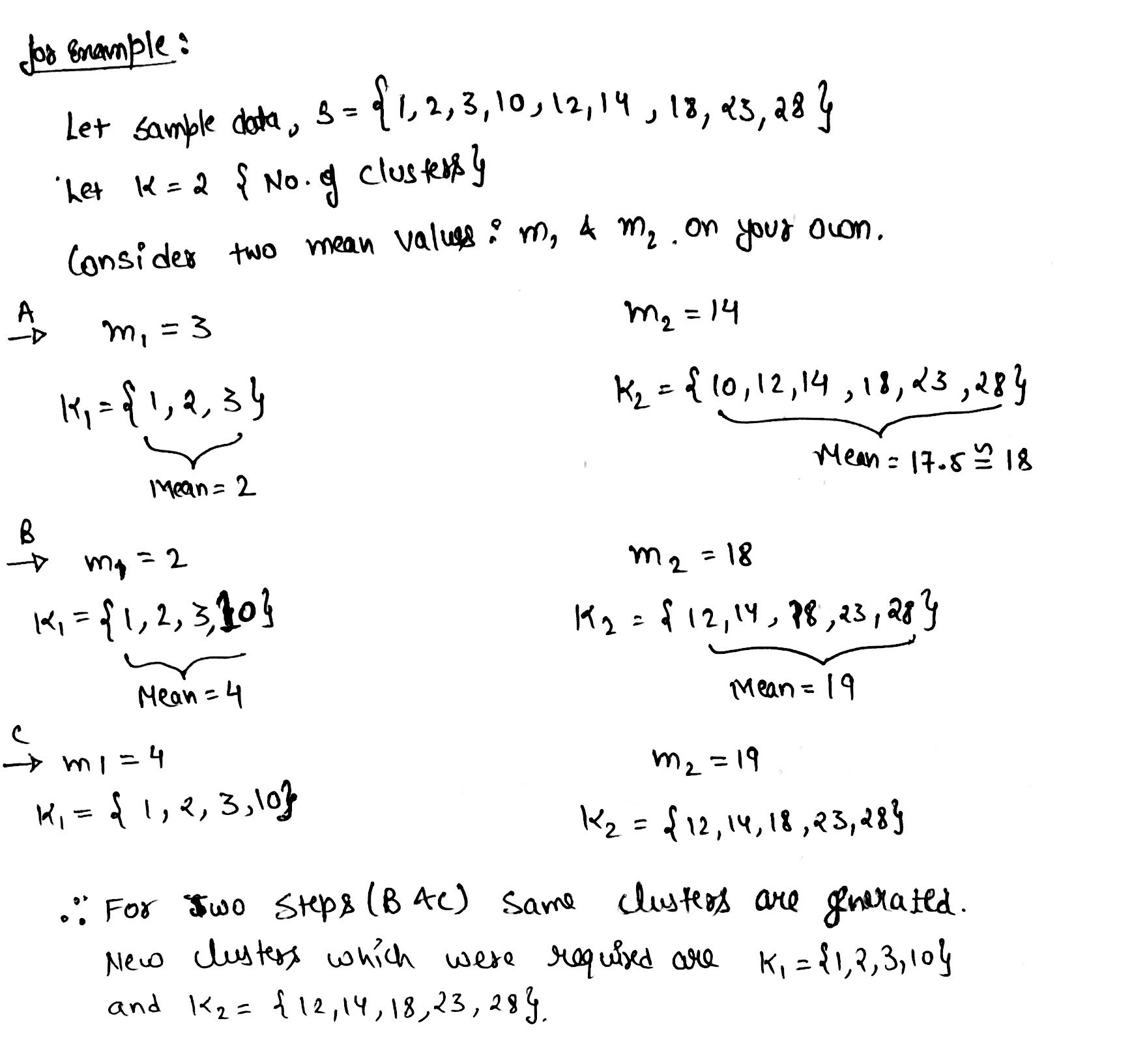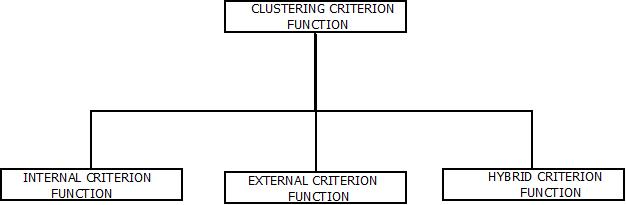# UNSUPERVISED LEARNING AND CLUSTERING

Unsupervised Learning & Clustering | Pattern Recognition Tutorial | Minigranth

# K-Means Clustering : Introduction

• K-Means clustering is known to be one of the simplest unsupervised learning algorithms that is capable of solving well known clustering problems.
• K-Means clustering algorithm can be executed in order to solve a problem using four simple steps:
• Make the partition of objects into K non empty steps i.e. K=1,2,3,.. .
• Consider arbitrary seed points from sample data.
• Calculate mean distance of sample data from seed points in order to generate clusters.
• Repeat the above steps until values of two clusters becomes same. Below is an solved example.Example : K-means Clustering

### Criterion Function : Clustering

• To measure the quality of clustering ability of any partitioned data set, criterion function is used.
• Consider a set , B = { x1,x2,x3…xn} containing “n” samples, that is partitioned exactly into “t” disjoint subsets i.e. B1, B2,…..,Bt.
• The main highlight of these subsets is, every individual subset represents a cluster.
• Sample inside the cluster will be similar to each other and dissimilar to samples in other clusters.
• To make this possible, criterion functions are used according the occurred situations.Criterion Function For Clustering

1. Internal Criterion Function
• This class of clustering is an intra-cluster view.
• Internal criterion function optimizes a function and measures the quality of clustering ability various clusters which are different from each other.
1. External Criterion Function
• This class of clustering criterion is an inter-class view.
• External Criterion Function optimizes a function and measures the quality of clustering ability of various clusters which are different from each other.
1. Hybrid Criterion Function
• This function is used as it has the ability to simultaneously optimize multiple individual Criterion Functions unlike as Internal Criterion Function and External Criterion Function